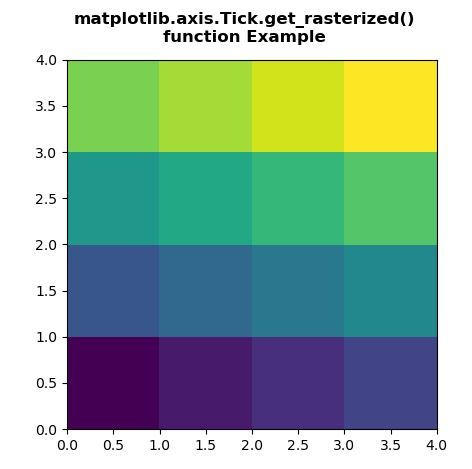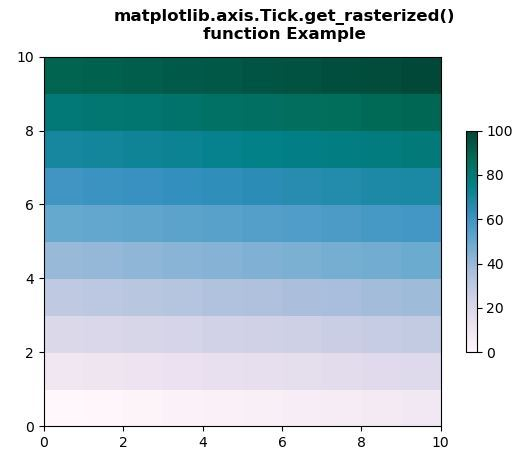# Matplotlib.axis.Tick.get_rasterized() in Python

Matplotlib is a library in Python and it is numerical – mathematical extension for NumPy library. It is an amazing visualization library in Python for 2D plots of arrays and used for working with the broader SciPy stack.

## matplotlib.axis.Tick.get_rasterized() Function

The Tick.get_rasterized() function in axis module of matplotlib library is used to get whether the artist is to be rasterized.

Syntax: Tick.get_rasterized(self)

Parameters: This method does not accepts any parameter.

Return value: This method return whether the artist is to be rasterized.

Below examples illustrate the matplotlib.axis.Tick.get_rasterized() function in matplotlib.axis:

Example 1:

## Python3

 `# Implementation of matplotlib function ` `from` `matplotlib.axis ``import` `Tick ` `import` `numpy as np ` `import` `matplotlib.pyplot as plt ` ` `  ` `  `d ``=` `np.arange(``16``).reshape(``4``, ``4``) ` `xx, yy ``=` `np.meshgrid(np.arange(``5``), np.arange(``5``)) ` ` `  `fig, ax ``=` `plt.subplots() ` ` `  `ax.set_aspect(``1``) ` `m ``=` `ax.pcolormesh(xx, yy, d) ` ` `  `if` `Tick.get_rasterized(m) ``=``=` `None``: ` `    ``Tick.set_rasterized(m, ``True``) ` ` `  `fig.suptitle(``"""matplotlib.axis.Tick.get_rasterized() ` `function Example\n"""``, fontweight``=``"bold") ` ` `  `plt.show() `

Output:Example 2:

## Python3

 `# Implementation of matplotlib function ` `from` `matplotlib.axis ``import` `Tick ` `import` `matplotlib.pyplot as plt ` `import` `matplotlib.colors as mcolors ` `import` `matplotlib.gridspec as gridspec ` `import` `numpy as np ` ` `  ` `  `arr ``=` `np.arange(``100``).reshape((``10``, ``10``)) ` `norm ``=` `mcolors.Normalize(vmin``=``0.``, vmax``=``100.``) ` ` `  `pc_kwargs ``=` `{``'cmap'``: ``'PuBuGn'``, ``'norm'``: norm} ` ` `  `fig, ax ``=` `plt.subplots() ` ` `  `m ``=` `ax.pcolormesh(arr, ``*``*``pc_kwargs) ` `fig.colorbar(m, ax``=``ax, shrink``=``0.6``) ` ` `  `if` `Tick.get_rasterized(m) ``=``=` `None``: ` `    ``Tick.set_rasterized(m, ``True``) ` ` `  `fig.suptitle(``"""matplotlib.axis.Tick.get_rasterized() ` `function Example\n"""``, fontweight``=``"bold") ` ` `  `plt.show() `

Output:Whether you're preparing for your first job interview or aiming to upskill in this ever-evolving tech landscape, GeeksforGeeks Courses are your key to success. We provide top-quality content at affordable prices, all geared towards accelerating your growth in a time-bound manner. Join the millions we've already empowered, and we're here to do the same for you. Don't miss out - check it out now!

Previous
Next